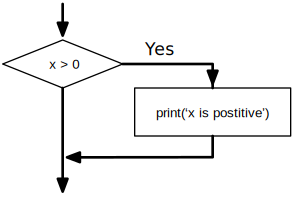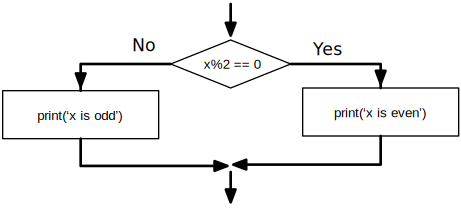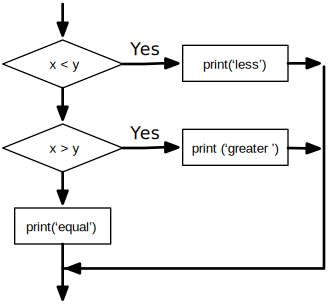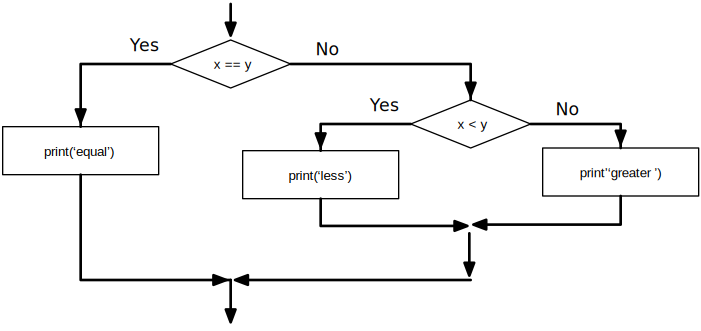# Conditional execution

## Boolean expressions

A boolean expression is an expression that is either true or false. The following examples use the operator ==, which compares two operands and produces True if they are equal and False otherwise:

True and False are special values that belong to the class bool; they are not strings:

>>> type(True)
<class 'bool'>
>>> type(False)
<class 'bool'>

The == operator is one of the comparison operators; the others are:

      x != y               # x is not equal to y
x > y                # x is greater than y
x < y                # x is less than y
x >= y               # x is greater than or equal to y
x <= y               # x is less than or equal to y
x is y               # x is the same as y
x is not y           # x is not the same as y

Although these operations are probably familiar to you, the Python symbols are different from the mathematical symbols for the same operations. A common error is to use a single equal sign (=) instead of a double equal sign (==). Remember that = is an assignment operator and == is a comparison operator. There is no such thing as =< or =>.

## Logical operators

There are three logical operators: and, or, and not. The semantics (meaning) of these operators is similar to their meaning in English. For example,

x > 0 and x < 10

is true only if x is greater than 0 and less than 10.

n%2 == 0 or n%3 == 0 is true if either of the conditions is true, that is, if the number is divisible by 2 or 3.

Finally, the not operator negates a boolean expression, so not (x > y) is true if x > y is false; that is, if x is less than or equal to y.

Strictly speaking, the operands of the logical operators should be boolean expressions, but Python is not very strict. Any nonzero number is interpreted as "true."

>>> 17 and True
True

This flexibility can be useful, but there are some subtleties to it that might be confusing. You might want to avoid it until you are sure you know what you are doing.

## Conditional execution

In order to write useful programs, we almost always need the ability to check conditions and change the behavior of the program accordingly. Conditional statements give us this ability. The simplest form is the if statement:

if x > 0 :
print('x is positive')

The boolean expression after the if statement is called the condition. We end the if statement with a colon character (:) and the line(s) after the if statement are indented.If Logic

If the logical condition is true, then the indented statement gets executed. If the logical condition is false, the indented statement is skipped.

if statements have the same structure as function definitions or for loops1. The statement consists of a header line that ends with the colon character (:) followed by an indented block. Statements like this are called compound statements because they stretch across more than one line.

There is no limit on the number of statements that can appear in the body, but there must be at least one. Occasionally, it is useful to have a body with no statements (usually as a place holder for code you haven't written yet). In that case, you can use the pass statement, which does nothing.

if x < 0 :
pass          # need to handle negative values!

If you enter an if statement in the Python interpreter, the prompt will change from three chevrons to three dots to indicate you are in the middle of a block of statements, as shown below:

>>> x = 3
>>> if x < 10:
...    print('Small')
...
Small
>>>

## Alternative execution

A second form of the if statement is alternative execution, in which there are two possibilities and the condition determines which one gets executed. The syntax looks like this:

if x%2 == 0 :
print('x is even')
else :
print('x is odd')

If the remainder when x is divided by 2 is 0, then we know that x is even, and the program displays a message to that effect. If the condition is false, the second set of statements is executed.If-Then-Else Logic

Since the condition must either be true or false, exactly one of the alternatives will be executed. The alternatives are called branches, because they are branches in the flow of execution.

## Chained conditionals

Sometimes there are more than two possibilities and we need more than two branches. One way to express a computation like that is a chained conditional:

if x < y:
print('x is less than y')
elif x > y:
print('x is greater than y')
else:
print('x and y are equal')

elif is an abbreviation of "else if." Again, exactly one branch will be executed.If-Then-ElseIf Logic

There is no limit on the number of elif statements. If there is an else clause, it has to be at the end, but there doesn't have to be one.

if choice == 'a':
elif choice == 'b':
print('Good guess')
elif choice == 'c':
print('Close, but not correct')

Each condition is checked in order. If the first is false, the next is checked, and so on. If one of them is true, the corresponding branch executes, and the statement ends. Even if more than one condition is true, only the first true branch executes.

## Nested conditionals

One conditional can also be nested within another. We could have written the three-branch example like this:

if x == y:
print('x and y are equal')
else:
if x < y:
print('x is less than y')
else:
print('x is greater than y')

The outer conditional contains two branches. The first branch contains a simple statement. The second branch contains another if statement, which has two branches of its own. Those two branches are both simple statements, although they could have been conditional statements as well.Nested If Statements

Although the indentation of the statements makes the structure apparent, nested conditionals become difficult to read very quickly. In general, it is a good idea to avoid them when you can.

Logical operators often provide a way to simplify nested conditional statements. For example, we can rewrite the following code using a single conditional:

if 0 < x:
if x < 10:
print('x is a positive single-digit number.')

The print statement is executed only if we make it past both conditionals, so we can get the same effect with the and operator:

if 0 < x and x < 10:
print('x is a positive single-digit number.')

## Catching exceptions using try and except

Earlier we saw a code segment where we used the input and int functions to read and parse an integer number entered by the user. We also saw how treacherous doing this could be:

>>> prompt = "What...is the airspeed velocity of an unladen swallow?\n"
>>> speed = input(prompt)
What...is the airspeed velocity of an unladen swallow?
What do you mean, an African or a European swallow?
>>> int(speed)
ValueError: invalid literal for int() with base 10:
>>>

When we are executing these statements in the Python interpreter, we get a new prompt from the interpreter, think "oops", and move on to our next statement.

However if you place this code in a Python script and this error occurs, your script immediately stops in its tracks with a traceback. It does not execute the following statement.

Here is a sample program to convert a Fahrenheit temperature to a Celsius temperature:

If we execute this code and give it invalid input, it simply fails with an unfriendly error message:

python fahren.py
Enter Fahrenheit Temperature:72
22.22222222222222
python fahren.py
Enter Fahrenheit Temperature:fred
Traceback (most recent call last):
File "fahren.py", line 2, in <module>
fahr = float(inp)
ValueError: could not convert string to float: 'fred'

There is a conditional execution structure built into Python to handle these types of expected and unexpected errors called "try / except". The idea of try and except is that you know that some sequence of instruction(s) may have a problem and you want to add some statements to be executed if an error occurs. These extra statements (the except block) are ignored if there is no error.

You can think of the try and except feature in Python as an "insurance policy" on a sequence of statements.

We can rewrite our temperature converter as follows:

Python starts by executing the sequence of statements in the try block. If all goes well, it skips the except block and proceeds. If an exception occurs in the try block, Python jumps out of the try block and executes the sequence of statements in the except block.

python fahren2.py
Enter Fahrenheit Temperature:72
22.22222222222222
python fahren2.py
Enter Fahrenheit Temperature:fred
Please enter a number

Handling an exception with a try statement is called catching an exception. In this example, the except clause prints an error message. In general, catching an exception gives you a chance to fix the problem, or try again, or at least end the program gracefully.

## Short-circuit evaluation of logical expressions

When Python is processing a logical expression such as x >= 2 and (x/y) > 2, it evaluates the expression from left to right. Because of the definition of and, if x is less than 2, the expression x >= 2 is False and so the whole expression is False regardless of whether (x/y) > 2 evaluates to True or False.

When Python detects that there is nothing to be gained by evaluating the rest of a logical expression, it stops its evaluation and does not do the computations in the rest of the logical expression. When the evaluation of a logical expression stops because the overall value is already known, it is called short-circuiting the evaluation.

While this may seem like a fine point, the short-circuit behavior leads to a clever technique called the guardian pattern. Consider the following code sequence in the Python interpreter:

>>> x = 6
>>> y = 2
>>> x >= 2 and (x/y) > 2
True
>>> x = 1
>>> y = 0
>>> x >= 2 and (x/y) > 2
False
>>> x = 6
>>> y = 0
>>> x >= 2 and (x/y) > 2
Traceback (most recent call last):
File "<stdin>", line 1, in <module>
ZeroDivisionError: division by zero
>>>

The third calculation failed because Python was evaluating (x/y) and y was zero, which causes a runtime error. But the second example did not fail because the first part of the expression x >= 2 evaluated to False so the (x/y) was not ever executed due to the short-circuit rule and there was no error.

We can construct the logical expression to strategically place a guard evaluation just before the evaluation that might cause an error as follows:

>>> x = 1
>>> y = 0
>>> x >= 2 and y != 0 and (x/y) > 2
False
>>> x = 6
>>> y = 0
>>> x >= 2 and y != 0 and (x/y) > 2
False
>>> x >= 2 and (x/y) > 2 and y != 0
Traceback (most recent call last):
File "<stdin>", line 1, in <module>
ZeroDivisionError: division by zero
>>>

In the first logical expression, x >= 2 is False so the evaluation stops at the and. In the second logical expression, x >= 2 is True but y != 0 is False so we never reach (x/y).

In the third logical expression, the y != 0 is after the (x/y) calculation so the expression fails with an error.

In the second expression, we say that y != 0 acts as a guard to insure that we only execute (x/y) if y is non-zero.

## Debugging

The traceback Python displays when an error occurs contains a lot of information, but it can be overwhelming. The most useful parts are usually:

• What kind of error it was, and

• Where it occurred.

Syntax errors are usually easy to find, but there are a few gotchas. Whitespace errors can be tricky because spaces and tabs are invisible and we are used to ignoring them.

>>> x = 5
>>>  y = 6
File "<stdin>", line 1
y = 6
^
IndentationError: unexpected indent

In this example, the problem is that the second line is indented by one space. But the error message points to y, which is misleading. In general, error messages indicate where the problem was discovered, but the actual error might be earlier in the code, sometimes on a previous line.

In general, error messages tell you where the problem was discovered, but that is often not where it was caused.

## Glossary

body
The sequence of statements within a compound statement.

boolean expression
An expression whose value is either True or False.

branch
One of the alternative sequences of statements in a conditional statement.

chained conditional
A conditional statement with a series of alternative branches.

comparison operator

One of the operators that compares its operands: ==, !=, >, <, >=, and <=.

conditional statement
A statement that controls the flow of execution depending on some condition.

condition
The boolean expression in a conditional statement that determines which branch is executed.

compound statement
A statement that consists of a header and a body. The header ends with a colon (:). The body is indented relative to the header.

guardian pattern
Where we construct a logical expression with additional comparisons to take advantage of the short-circuit behavior.

logical operator

One of the operators that combines boolean expressions: and, or, and not.

nested conditional
A conditional statement that appears in one of the branches of another conditional statement.

traceback
A list of the functions that are executing, printed when an exception occurs.

short circuit
When Python is part-way through evaluating a logical expression and stops the evaluation because Python knows the final value for the expression without needing to evaluate the rest of the expression.

## Exercises

Exercise 1: Rewrite your pay computation to give the employee 1.5 times the hourly rate for hours worked above 40 hours.

Enter Hours: 45
Enter Rate: 10
Pay: 475.0

Exercise 2: Rewrite your pay program using try and except so that your program handles non-numeric input gracefully by printing a message and exiting the program. The following shows two executions of the program:

Enter Hours: 20
Enter Rate: nine
Error, please enter numeric input
Enter Hours: forty
Error, please enter numeric input

Exercise 3: Write a program to prompt for a score between 0.0 and 1.0. If the score is out of range, print an error message. If the score is between 0.0 and 1.0, print a grade using the following table:

Score   Grade
>= 0.9     A
>= 0.8     B
>= 0.7     C
>= 0.6     D
< 0.6    F
~~~


Enter score: 0.95 A ~~

Enter score: perfect
Bad score
Enter score: 10.0
Bad score
Enter score: 0.75
C
Enter score: 0.5
F

Run the program repeatedly as shown above to test the various different values for input.

1. We will learn about functions in Chapter 4 and loops in Chapter 5.